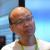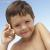## 关于C语言中的输入问题

```#include <stdio.h>

main()
{
char ch;

scanf("%c",&ch);
printf("%d\n",ch);

}```

```void InitInput()                 /* 清空输入缓存 */
{
char ch;
do {
p = getchar();
} while (ch != '\n');
} ```

0

0#### 引用来自“王小泉”的答案

000```while(getchar() != '\n')
;```
0#### 引用来自“yangjingwei”的答案

0```#include <stdio.h>
#include <string.h>

void InitInput()                 /* 清空输入缓存 */
{
while (getchar() != '\n');
}

main()
{
char ch;

scanf("%s",ch);
printf("%d\n",ch);       //为了显示换行符，故意使用%d
//	InitInput();
scanf("%s",ch);
printf("%d\n",ch);

}```
0```        char c;
c = getchar();
printf("%d\n", c);
InitInput();
c = getchar();
printf("%d\n", c);

```
0```#include <stdio.h>
#include <string.h>

char Input()
{
char ch;

ch = getchar();
if(ch != '\n')
{
while (getchar() != '\n');
}

return ch;
}

main()
{
char p;
p = Input();